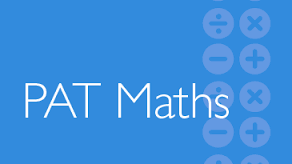Pat Maths (Booklet 5) (Pre Test)

39 Questions | Total Attempts: 440SettingsYEAR 7 (Feb) 2016.

• 1.
Jeremy's height is 1.7 meters.  Anna's height is 1.63 meters.What is the difference in their height?
• A.

4cm

• B.

7cm

• C.

13cm

• D.

56

• E.

64

• 2.
Tarek has \$5.00 to buy a kilogram of  'Koala'  biscuits. Which will give him the most change?
• A.

One 1 Kg pack

• B.

Two 500 g pack

• C.

Two 250 g packs and one 500 g pack

• D.

Four 250 g packs

• E.

He will get the same change no matter which may he chooses the kilograms

• 3.
Which percentage is equal to 0.49?
• A.

490%

• B.

49%

• C.

4.9%

• D.

049%

• 4.
Which number is larger than 0.17?
• A.

0.025

• B.

0.15

• C.

0.02

• D.

0.3

• E.

0.017

• 5.
Sue bought 5 bubblegums for 35 cents each. She gave the shopkeeper two dollars.How much change should she get?
• A.

25 cents

• B.

35 cents

• C.

50 cents

• D.

\$1.50

• E.

\$1.75

• 6.
6.07 million may be written as:
• A.

607 000 000

• B.

60 700 000

• C.

6 070 000

• D.

607 000

• 7.
Which set of cards have the numbers in order from smallest to largest?
• A.
• B.
• C.
• D.
• E.
• 8.
Which one of these numbers is 'five thousandths'?
• A.

5000

• B.

0.5000

• C.

0.005

• D.

0.0005

• 9.
• A.

20

• B.

22

• C.

24

• D.

30

• E.

None of these

• 10.
• A.

(R,3) and (S,3)

• B.

S,3) and (S,4)

• C.

(S,3) and (U,4)

• D.

(U,3) and (U,4)

• 11.
• A.

5000 centimetres

• B.

200 metres

• C.

500 metres

• D.

2 kilometres

• E.

5 kilometres

• 12.
• A.

WALRUS

• B.

WHALES

• C.

MONKEY

• D.

MANTIS

• E.

DONKEY

• 13.
• A.

Square

• B.

Parallelogram

• C.

Rectangle

• D.

Hexagon

• E.

Triangle

• 14.
Which of these polygons cannot be drawn?
• A.

A triangle with 2 sides parallel

• B.

A pentagon with 2 sides parallel

• C.

A quadrilateral with 2 pairs of parallel sides

• D.

A hexagon with 3 pairs of parallel sides

• E.

They can all be drawn

• 15.
• A.

A half turn in an anticlockwise direction

• B.

A half turn in a clockwise direction

• C.

A right angle turn in an anticlockwise direction

• D.

A right angle turn in a clockwise direction

• 16.
Use the scale on this map.Which point is 3.5 meters from point X?
• A.

P

• B.

Q

• C.

R

• D.

S

• E.

T

• 17.
Nina's plane landed at 7.43pm. What time is this in 24-hour time?
• A.

0543

• B.

0743

• C.

1443

• D.

1743

• E.

1943

• 18.
A sack of flour that weighs 4.5 kilograms has 300 grams taken from it.What does the sack weigh now?
• A.

1.5 kg

• B.

4.2 kg

• C.

4.47 kg

• D.

2.55 kg

• E.

29.5 kg

• 19.
The time shown on this clock is:
• A.

Twenty-five past ten

• B.

Five to five

• C.

Six to five

• D.

Six to six

• E.

Six to four

• 20.
• A.

24 units

• B.

28 units

• C.

36 units

• D.

44 units

• E.

48 units

• 21.
• A.

3 m

• B.

4 m

• C.

5 m

• 22.
• A.

12 units

• B.

18 units

• C.

24 units

• D.

30 units

• E.

36 units

• 23.
The first 20 kilometres took Helen:
• A.

• B.

• 24.
How far did Helen ride in the second hour?
• A.

• B.

• C.

• D.

• 25.
The graph shows that between 3:30 and 4:30. Helen:
• A.

Rode at constant speed

• B.

Rode on flat ground

• C.

Rode in an easterly direction

• D.

Stopped riding

Related TopicsBack to top